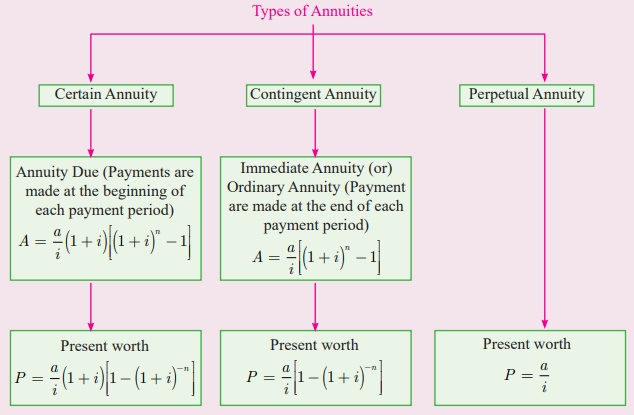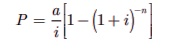Home | | Business Maths 11th std | Summary of Financial Mathematics

# Summary of Financial Mathematics

Business Mathematics and Statistics(EMS) : Financial MathematicsEndowment or Scholarship fund

If the scholarship is awarded endlessly, then P =a/i

If the scholarship is awarded for a fixed period, say, n years, thenFace Value: The original Value of the share is called its nominal values or face value or printed value.

Market Value: The price at which the share is sold (or) purchased in the capital market through stock exchanges is called the market value.

A Share is called at par if the market value of the share is equal to its face (or) nominal value.

A share is said to be above par (or) at premium, if the market value of the share is more than its nominal value.

A share is said to be below par (or) at discount, if the market value of the share is less than its nominal value.

The part of the annual profit, which a share holder gets for his investment from the company is called dividend.

Dividend is always declared on the face value of the share and the rate of dividend is expressed as a percentage of the nominal value of a share per annum.

Annual income of a shareholders = n×r×F.V  / 100

Where n = number of shares with the shareholders

r = rate of dividend,

Annual Return = Annual income / investment in shares ×100%

Number of Shares held = investment  / M.V (or) F.V. of one share (as the type of investment)

(or)

= Annual income / income from one share

(or) = Total F.V / F.V. of one share

Which is better investment for two stocks

Let the investment each case be = (M.V of First stock × M.V. of Second Stock)

Case (i)

Income form r1 % = [ r1 /  (investment in each stock)  ] × Total investment

Case (ii)

Income form r2 % = [ r2 / (investment in each stock) ] ×Total investment

Tags : Financial Mathematics , 11th Business Mathematics and Statistics(EMS) : Chapter 7 : Financial Mathematics
Study Material, Lecturing Notes, Assignment, Reference, Wiki description explanation, brief detail
11th Business Mathematics and Statistics(EMS) : Chapter 7 : Financial Mathematics : Summary of Financial Mathematics | Financial Mathematics